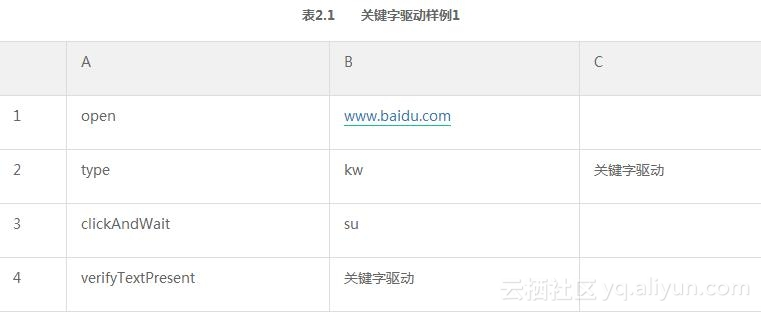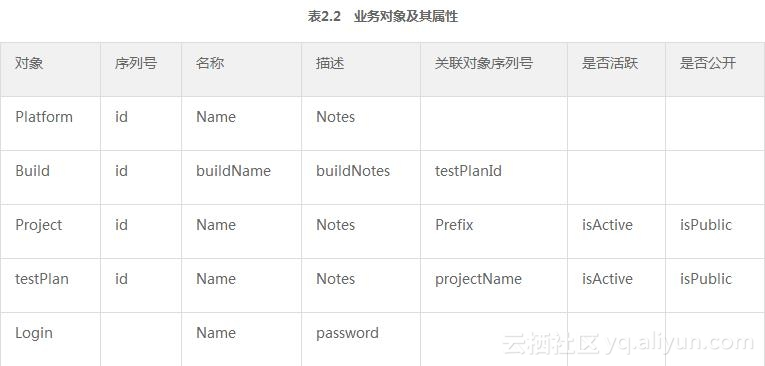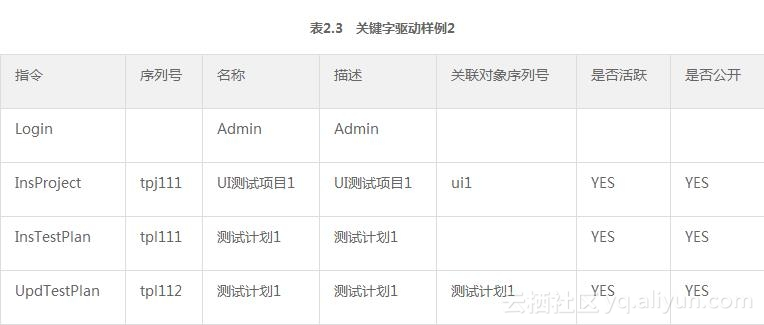#《精通自动化测试框架设计》—第2章 2.5节使用Exce

0
0
01. 云栖社区>
2. 博客>
3. 正文

## 《精通自动化测试框架设计》—第2章 2.5节使用Exce

2.5　使用Excel
2.5.1　经典的DataTable（1）如有可能，使用与开发团队相同的语言、IDE（集成开发环境）来构建自动化测试框架，编写用例，甚至复用其代码评审工具与版本集成环境。

（2）如有可能，让编写自动化测试用例的工程师参与编码，甚至是参与部分框架搭建的机会，这会成为整个自动化项目成功的有力保障之一。

2.5.2　强关键字驱动的自动化用例

（1）拓展目标用户：将该套框架的目标使用者从传统的系统测试工程师往前推进到敏捷中的接收测试（Acceptance Test）或者用户验收测试（User Acceptance Testing）参与者之中。也就是让产品经理（Product Owner）或者用户代表等代表领域专家（Domain Expert）作为自动化测试的目标用户。（2）创建测试项目（序列号：tpj111,名称……略）。

（3）创建测试计划（序列号：tp111,名称……略）。

（4）修改测试计划，将前述创建的测试项目与之关联。2.5.3　Apache POI介绍

Microsoft Office是一个产品族，涵盖了这种类型的文档格式，POI也提供了不同的API组件来进行相应的支持。

Excel (SS=HSSF+XSSF)
Word (HWPF+XWPF)
PowerPoint (HSLF+XSLF)
OpenXML4J (OOXML)
OLE2 Filesystem (POIFS)
OLE2 Document Props (HPSF)
Outlook (HSMF)
Visio (HDGF)
TNEF (HMEF)
Publisher (HPBF)


2.5.4　单个工作表的解析
Excel工作簿是由一个个工作表所组成的。很多情况下当讨论如何进行Excel解析的时候，其实是在讨论如何解析其中的一个工作表。

package com.dataset.excel;

import java.util.ArrayList;
import java.util.List;
import org.apache.poi.ss.usermodel.Cell;
import org.apache.poi.ss.usermodel.DateUtil;
import org.apache.poi.ss.usermodel.Row;
import org.apache.poi.ss.usermodel.Sheet;

public class SheetData {
private Sheet sheet;
private List< String[] > dataList = new ArrayList< String[] >();
public SheetData(Sheet sheet){
this.sheet=sheet;
setSheetDataSet();
}
protected　String getCellText (Row row,int column) {
String cellText="";
Cell cell = row.getCell(column, Row.CREATE_NULL_AS_BLANK);
switch(cell.getCellType()){
case Cell.CELL_TYPE_STRING:
cellText = cell.getStringCellValue().trim();
break;
case Cell.CELL_TYPE_BLANK:
cellText = "";
break;
case Cell.CELL_TYPE_BOOLEAN:
cellText = Boolean.toString(cell.getBooleanCellValue());
break;
case Cell.CELL_TYPE_NUMERIC:
if(DateUtil.isCellDateFormatted(cell)){
cellText = String.valueOf(cell.getDateCellValue());
}else{
cell.setCellType(Cell.CELL_TYPE_STRING);
String value= cell.getStringCellValue();
if(value.indexOf(".") >-1){
cellText = String.valueOf(new Double(value)).trim();
}else{
cellText = value.trim();
}
}
break;
case Cell.CELL_TYPE_ERROR:
cellText = "";
break;
case Cell.CELL_TYPE_FORMULA:
cell.setCellType(Cell.CELL_TYPE_STRING);
cellText = cell.getStringCellValue();
if(cellText!=null){
cellText = cellText.replaceAll("#N/A","").trim();
}
break;
default:
cellText = "";
break;
}
return cellText;
}
private void setSheetDataSet(){
int columnNum = 0;
if(sheet.getRow(0)!=null){
columnNum = sheet.getRow(0).getLastCellNum()-sheet.getRow(0).
getFirstCellNum();
}
if(columnNum >0){
for(Row row:sheet){
String[] singleRow = new String[columnNum];
int n = 0;
for(int i=0;i< columnNum;i++){
singleRow[n]=this.getCellText(row, i);
n++;
}
if("".equals(singleRow)){continue;}
}
}
}
public List< String[] > getSheetDataSet(){
return dataList;
}
public int getRowCount(){
return sheet.getLastRowNum();
}
public int getColumnCount(){
Row row = sheet.getRow(0);
if(row!=null&&row.getLastCellNum() >0){
return row.getLastCellNum();
}
return 0;
}

public String[] getRowData(int rowIndex){
String[] dataArray = null;
if(rowIndex >this.getRowCount()){
return dataArray;
}else{
dataArray = new String[this.getColumnCount()];
return this.dataList.get(rowIndex);
}

}
public String[] getColumnData(int colIndex){
String[] dataArray = null;
if(colIndex >this.getColumnCount()){
return dataArray;
}else{
if(this.dataList!=null&&this.dataList.size() >0){
dataArray = new String[this.getRowCount()+1];
int index = 0;
for(String[] rowData:dataList){
if(rowData!=null){
dataArray[index] = rowData[colIndex];
index++;
}
}
}
}
return dataArray;

}

public String getText(int row, int column){
return getRowData(row)[column];
}
}


private Sheet sheet;
private List< String[] > dataList = new ArrayList< String[] >();

public SheetData(Sheet sheet){
this.sheet=sheet;
setSheetDataSet();
}


Sheet类是POI包中有关工作表的对象模型。整个SheetData也可以说是在Sheet类之上又进行了一层封装，提供了一些更为适用于本次案例的工作表数据读取方法。

singleRow[n]=this.getCellText(row, i);

switch(cell.getCellType())

public String[] getRowData(int rowIndex)
public String[] getColumnData(int colIndex)


2.5.5　整个工作簿的解析

package com.dataset.excel;
import java.io.IOException;
import java.io.InputStream;
import java.util.HashMap;
import org.apache.poi.openxml4j.exceptions.InvalidFormatException;
import org.apache.poi.ss.usermodel.Workbook;
import org.apache.poi.ss.usermodel.WorkbookFactory;

public class ExcelPaser {
private Workbook wb = null;
private HashMap< String,SheetData > sheetDataMap=new HashMap< String,SheetData >();
private int sheetNum=-1;
public ExcelPaser(InputStream in){
try {
wb = WorkbookFactory.create(in);
sheetNum=　wb.getNumberOfSheets();
setSheetDataMap();
}　catch (InvalidFormatException e) {
e.printStackTrace();
} catch (IOException e) {
e.printStackTrace();
}

}
private void setSheetDataMap(){
for(int sheetIndex=0;sheetIndex< sheetNum;sheetIndex++){
String sheetName=wb.getSheetName(sheetIndex);
SheetData sheetData= new SheetData(wb.getSheetAt(sheetIndex));
sheetDataMap.put(sheetName, sheetData);
}
}

public SheetData getSheetDataByName(String sheetName){
return sheetDataMap.get(sheetName);
}
public int getSheetIndex(String sheetName){
int index=wb.getSheetIndex(sheetName);
//　System.out.println("\nsheet["+index+"]="+sheetName);
return index ;
}
public String getText(String sheetName,int row, int column){
return getSheetDataByName(sheetName).getText(row, column);

}

}


public ExcelPaser(InputStream in){
try {
wb = WorkbookFactory.create(in);
sheetNum=　wb.getNumberOfSheets();
setSheetDataMap();
}　//其余略
}


SheetData sheetData= new SheetData(wb.getSheetAt(sheetIndex));

2.5.6　用例解析与执行

package com.dataset.excel;

import java.io.FileInputStream;
import java.io.FileNotFoundException;
import java.io.InputStream;
import java.lang.reflect.Method;
import java.util.Arrays;
import java.util.HashMap;
import java.util.Map;
import org.apache.poi.ss.usermodel.Sheet;
import com.dataset.excel.MockAPI.MockAPI;

static {
}
private static SheetData sheetData;
String path="sample.xls";
String sheetName="用例111"; //实际项目中这两个可来自配置文件
long now=System.currentTimeMillis();
try {
InputStream in = new FileInputStream(path);
} catch (FileNotFoundException e) {
e.printStackTrace();
}
long delta=System.currentTimeMillis()-now;
System._b>out__.println("\n Time used in ms::"+delta);
}
}

public static String[] getCallList(){
return sheetData.getColumnData(0);
}

public static String[] getParams(int rowIndex){
return sheetData.getRowData(rowIndex);
}

public static void main(String args[]){
Class< ? > clazz = MockAPI.class;
Method[] methods =clazz.getDeclaredMethods();
String [] callList=getCallList();
for(int i=1;i< callList.length;i++){
for(Method m:methods){
if( m.getName().equalsIgnoreCase(callList[i])){
try {
m.invoke(new MockAPI(),(Object)getParams(i));
} catch (Exception ex) {
ex.printStackTrace();
}
}
}
}
}
}


static {
}


String path="sample.xls";
String sheetName="用例111";


sheetData=　reader.getSheetDataByName(sheetName);


for(int i=1;i< callList.length;i++)


Class< ? > clazz = MockAPI.class;
Method[] methods =clazz.getDeclaredMethods();

for(Method m:methods){
if( m.getName().equalsIgnoreCase(callList[i])){
try {
m.invoke(new MockAPI(),(Object)getParams(i));
} //略
}


long now=System.currentTimeMillis();

Done to parse sheet '用例111' in ms::731
************************************
**Matched Method::InsProject
**************called InsProject*****
Params::{isActive=ui1, prefix=UI测试项目1, name=tpj111, isPublic=YES, notes=UI测试项目1}


Done to parse sheet '用例111' in ms::1350


Done to parse sheet '用例111' in ms::2462


2.5.7　Mock API类

package com.dataset.excel.MockAPI;
import java.util.Map;
public boolean login(Map < String,String > params){
System.out.println("Params::"+params.toString());
System.out.println("************************************");
return true;
}
public void InsProject(Map < String,String > params){
System.out.println("**************called InsProject*****");
System.out.println("Params::"+params.toString());
System.out.println("************************************");
}
}


2.5.8　Mock API适配器类

package com.dataset.excel.MockAPI;
import java.util.HashMap;
import java.util.Map;
public class MockAPI {
public static final String[]　InsProjectArray={"id","name","notes","prefix",
"isActive","isPublic"};

if (map!=null){
}
else return false;
}
public void InsProject(String [] valuses){
Map< String,String > map =getParamMap(InsProjectArray,valuses);
if (map!=null){
api.InsProject(map);
}
}

private Map< String,String >getParamMap(String[] params,String[] valuses ){
if (params.length >valuses.length){
System.out.println("value number is less than param number");
return null;
}
Map< String,String > map=new HashMap< String,String >();
for(int i=1;i< params.length;i++){
if(params[i]!=""){
map.put(params[i], valuses[i]);
}
}
return map;
}
}


+ 关注